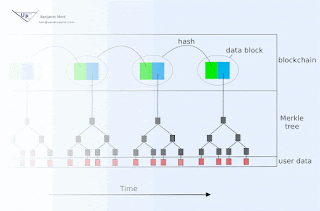## Wednesday, November 8, 2017

### DCKR vulnerable to time traveling cipherpunks

Conventional asymmetric cryptosystems assume computational hardness of this or that math problem (e.g. factoring, discrete logarithm, R-LWE, etc). The lack of proof of classical and quantum hardness of these math problems is what gives me pause, and is much of why I propose DCKR.

What assumption then does DCKR make for derivation of asymmetry? It assumes time travel is not possible. If anyone invents a time machine, we might have to reassess utility of DCKR as well.

(DCKR further relies on a cryptographic hash function, so irreversibility of that hash function is also necessary. But we generally rely on existence of such a function anyhow, so the goal is to not introduce additional attack points into such systems. Also, we have a larger number of hash functions to choose from - so we can more readily swap out one function for another should a vulnerability be found.)